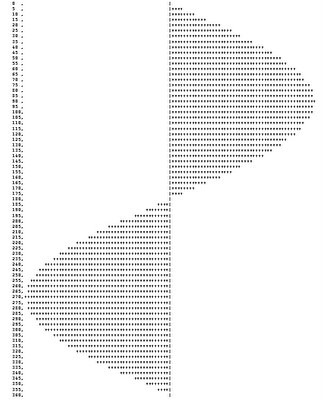## Oct 15, 2006

### A Scenario to Ponder #4

This scenario doesn't (and hopefully shouldn't) relate to any requirements, you will ever see, to be implemented at the database tier. But just for the fun of solving it, I am putting it here.
Please have a look at the image. Its a sine curve, that is generated from a query selecting 2 columns (both computed, may be from number table). One column gives the angle in degrees and the other column is the corresponding sine value, graphically represented.

The result set should have values of the angle from 0 to 360 in steps of 5. And lets say the scale of the graph is 50 (meaning you will have 50 '+' for the sine value = 1). The output given below should give a better insight.Can anyone come up with the query? The solution can be in SQL Server 2000 or 2005.

Anonymous said...

Easy, mate (assume I have a table - nums (num int), populated to at least 361)

select
right(space(3) + cast(num-1 as varchar(3)),3),
right(space(50) + case when sin(radians(num-1.)) < 0 then replicate('+',50 * -sin(radians(num-1.))) else '' end,50)
+ '|' +
left(case when sin(radians(num-1.)) > 0 then replicate('+',50 * sin(radians(num-1.))) else '' end + space(50),50)
from dbo.nums
where (num-1) % 5 = 0
and num <= 361

Omnibuzz said...

Thats absolutely right Rob :)
And you can get the Cosine curve too by just changing the function.
Good show!!

-Omni

Omnibuzz said...

Here is my solution:
Fairly the same idea as Rob, but I don't use the nums table, I use CTEs to generate the numbers.

-- Sine Graph
with SineGraph as
(
select 0 as val,0 as sinval
union all
select val + 5,cast(sin(radians(val + 5.0))*50 as int) from SineGraph where val < 360
)
select cast(val as char(3)) as degrees,reverse(cast(isnull(replicate('+',-sinval),'') as char(50))) + '|' + isnull(replicate('+',sinval),'') as Graph from SineGraph;

And here are the queries to generate the Cosine and Tan graphs

-- Cos Graph
with CosGraph as
(
select 0 as val,50 as cosval
union all
select val + 5,cast(cos(radians(val + 5.0))*50 as int) from CosGraph where val < 360
)
select cast(val as char(3)) as degrees,reverse(cast(isnull(replicate('+',-cosval),'') as char(50))) + '|' + isnull(replicate('+',cosval),'') as Graph from CosGraph;

-- Tan Graph
with TanGraph as
(
select 0 as val,cast(0 as bigint) as tanval
union all
select val + 5,cast(ceiling(tan(radians(val + 5.0))*5) as bigint)from TanGraph where val < 360
)
select cast(val as char(3)) as degrees,case when (val + 90) %180 = 0 then replicate('-',123) else reverse(cast(isnull(replicate('+',-tanval),'') as char(60))) + '|' + isnull(replicate('+',tanval),'') end as Graph from TanGraph

Anonymous said...

Thats really amazing ...

Thanks
Prateek Agrawal

Unknown said...

sir any one please tell me how to insert records automatically upto number which we like

in above example create a nums table and it contain 361 records

without typing 361 times can i insert in single time

is it possible

help me

--------------------

maild id: mganeshm143@gmail.com

=------------------